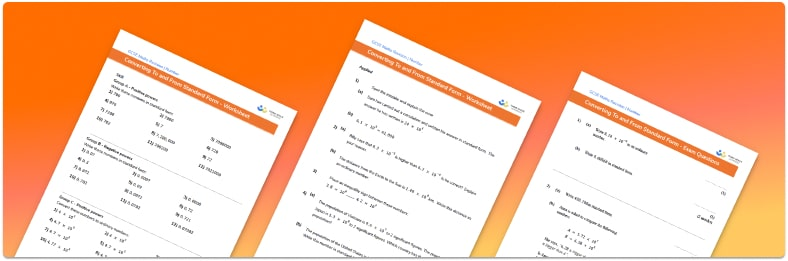# Converting To And From Standard Form WorksheetHelp your students prepare for their Maths GCSE with this free Converting To and From Standard Form worksheet of 68 questions and answers

• Section 1 of the Converting To and From Standard Form worksheet contains 12 skills-based Converting To and From Standard Form questions, in 5 groups to support differentiation
• Section 2 contains 4 applied Converting To and From Standard Form questions with a mix of worded problems and deeper problem solving questions
• Section 3 contains 4 foundation and higher level GCSE exam style Converting To and From Standard Form questions
• Answers and a mark scheme for all Converting To and From Standard Form questions
• Follows variation theory with plenty of opportunities for students to work independently at their own level
• All questions created by fully qualified expert secondary maths teachers
• Suitable for GCSE maths revision for AQA, OCR and Edexcel exam boards

• This field is for validation purposes and should be left unchanged.

You can unsubscribe at any time (each email we send will contain an easy way to unsubscribe). To find out more about how we use your data, see our privacy policy.

### Converting to and from standard form at a glance

Standard form is a simplified way of writing numbers. Standard form is most often used to write very large numbers or very small numbers, as it can make these numbers easier to read. When writing a number in standard form, we write it as a decimal place between 1 and 10, then multiplied by powers of ten. Numbers that are not written in standard form are referred to as ordinary numbers.

To convert any number to standard form, first write any significant figures as a decimal between 1 and 10. In order to  maintain the place value of the number we then multiply the decimal by a power of 10. For example, take the number 52300. In this number 5, 2 and 3 are significant figures, therefore the decimal is 5.23. To retain the place value of 52300, we need to multiply 5.23 by 10000 or 104. Therefore, 52300 is written as 5.23×104. The power of 10 will be negative if we are writing really small numbers.

Standard form numbers can be converted to ordinary numbers by multiplying the decimal by the power of 10.

Sometimes a number might look like it is in standard form as it is a number multiplied by a power of 10. However, it is important that the decimal in standard form is between 1 and 10. We can convert numbers written in this way to standard form by adjusting the place value of the decimal and the power of 10 accordingly.

Looking forward, students can then progress to additional number worksheets, for example aor aFor more teaching and learning support on Number our GCSE maths lessons provide step by step support for all GCSE maths concepts.

## Do you have KS4 students who need more focused attention to succeed at GCSE?There will be students in your class who require individual attention to help them succeed in their maths GCSEs. In a class of 30, it’s not always easy to provide.

Help your students feel confident with exam-style questions and the strategies they’ll need to answer them correctly with our dedicated GCSE maths revision programme.

Lessons are selected to provide support where each student needs it most, and specially-trained GCSE maths tutors adapt the pitch and pace of each lesson. This ensures a personalised revision programme that raises grades and boosts confidence.

Find out more